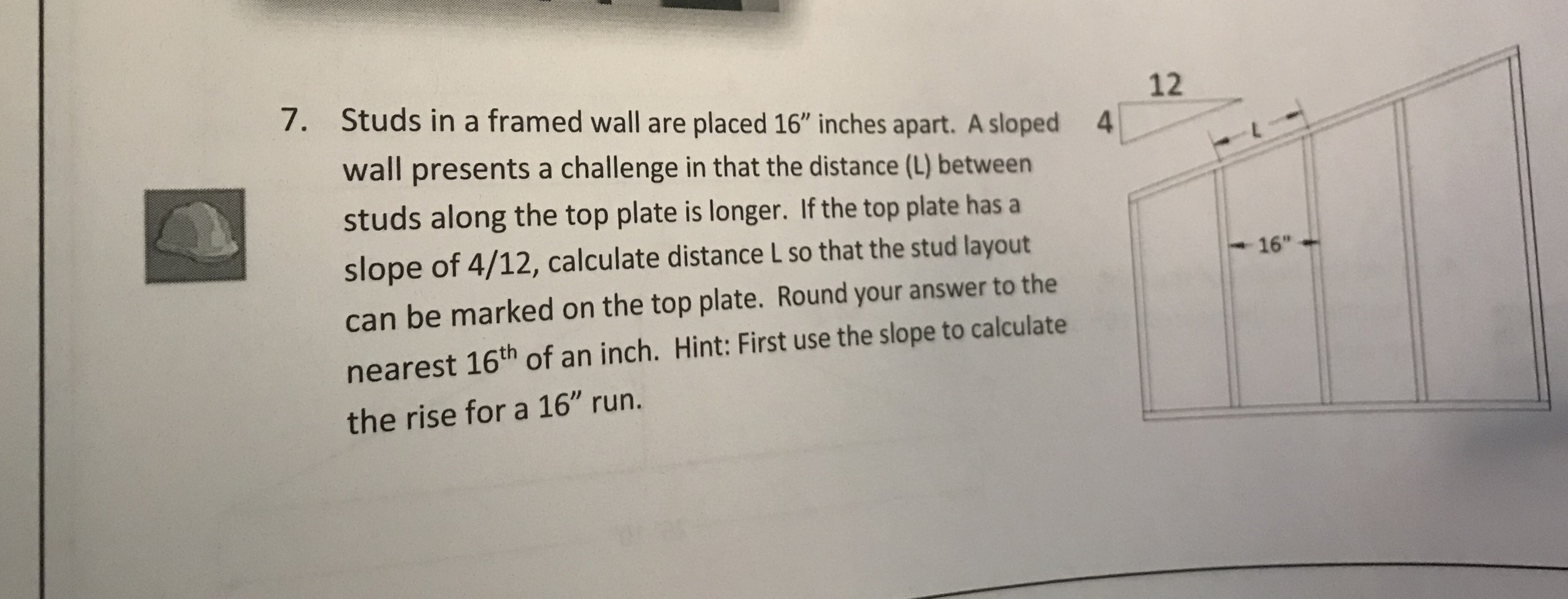7.12Studs in a framed wall are placed 16" inches apart. A slopedwall presents a challenge in that the distance (L) betweenstuds along the top plate is longer. If the top plate has aslope of 4/12, calculate distance L so that the stud layoutcan be marked on the top plate. Round your answer to thenearest 16th of an inch. Hint: First use the slope to calculatethe rise for a 16" run.416"

Question

Please write detail explaination, approach and so onhelp_outlineImage Transcriptionclose7. 12 Studs in a framed wall are placed 16" inches apart. A sloped wall presents a challenge in that the distance (L) between studs along the top plate is longer. If the top plate has a slope of 4/12, calculate distance L so that the stud layout can be marked on the top plate. Round your answer to the nearest 16th of an inch. Hint: First use the slope to calculate the rise for a 16" run. 4 16" fullscreen
Step 1

Given that Studs framed in a wall are 16'' apart.

Length of the sloped wall along the  top plate = L.

Slope of top plate = 4/12.

So here below a triangle ABC is formed where AB is distance between studs = 16 inch and AC is L distance along top plate. Let us assume x be the side BC.

Step 2

Given slope of top plate is 4/12.

According to the traingle formed Slope = opposite side/adjacent side = x...

Want to see the full answer?

See Solution

Want to see this answer and more?

Our solutions are written by experts, many with advanced degrees, and available 24/7

See Solution
Tagged in

Triangles